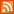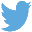Bucaro TecHelpHTTPS Encryption not required because no account numbers orpersonal information is ever requested or accepted by this siteCustom Search# Java Script Number Object

Normally a "number" is just a variable that you assigned a numerical value to. But JavaScript provides a Number object that gives you some powerful formatting routines for working with numbers.

Number Object Methods

 Method Returns toExponential(digits) A string containing the number in exponential form with digits specifying how many digits to the right of the decimal point should be returned. toFixed(digits) A string containing the number in fixed point form with digits specifying how many digits to the right of the decimal point should be returned. toLocaleString() A string that conforms to the client browser toPrecision(digits) A string in exponential or fixed point form with digits specifying how many digits should be returned. toString(radix) Returns the number as a string. The default radix parameter (10) converts the value to base-10 notation, or you can specify 2 for binary or 16 for hexadecimal to convert it to that base. valueOf() Returns the value of the number contained by the Number object

To demonstrate the use of the Number object along with some Math object methods, lets calculate a very large number, for example the volume of the earth in cubic miles. The equation for the volume of a sphere is shown below.

volume = 4 pi r ^ 3 / 3

Note: Our volume of the earth result using this equation will not be perfectly accurate because the earth is a not a perfect sphere, its flattened at the poles. For our calculation we will use the radius of the earth at the equator, 3963.19 miles.

First we'll use the Math.pow method to cube or raise the power of the radius to the third power as shown below.

```Math.pow(3963.19,3)
```

We plug that into a statement that calculates the numerator for the right side of our equation, as shown below.

```4 * Math.PI * (Math.pow(3963.19,3)
```

Note that we are using the Math.PI property to provide us with the value of pi.

Next we divide the numerator by the denominator, 3, as shown below.

```4 * Math.PI * (Math.pow(3963.19,3))/3
```

We create a Number object and initialize it with the result of this calculation, as shown below.

```var volume = new Number(4*Math.PI*(Math.pow(3963.19,3))/3);
```

If we write the result of this calculation as it stands, the value is incomprehensible, as shown below.

```260749383073.77307
```

 .menubtn { margin-top:10px; margin-left:50px; width:220px; opacity:1; border-style:solid; border-color:#009000; background-color:#66ff66; transition-property: opacity; transition-duration: 1s; transition-timing-function: ease-out; } .menubtn:hover { opacity:1; background-color:#e5ff23; } Menu - More Java Script Quick ReferenceRSS FeedFollow @Stephen Bucaro[Site User Agreement] [Privacy Policy] [Site map] [Search This Site] [Contact Form]
Copyright©2001-2023 Bucaro TecHelp 13771 N Fountain Hills Blvd Suite 114-248 Fountain Hills, AZ 85268